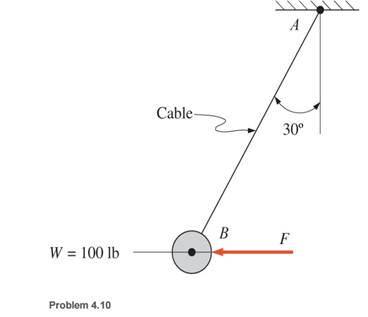### Create an Account

Home / Questions / Calculate the horizontal force F that should be applied to the 100 lb weight shown so that...

# Calculate the horizontal force F that should be applied to the 100 lb weight shown so that the cable AB is inclined at an angle of 30° with the vertical

Calculate the horizontal force F that should be applied to the 100 lb weight shown so that the cable AB is inclined at an angle of 30° with the vertical.Jun 26 2020 View more View LessSubscribe To Get Solution